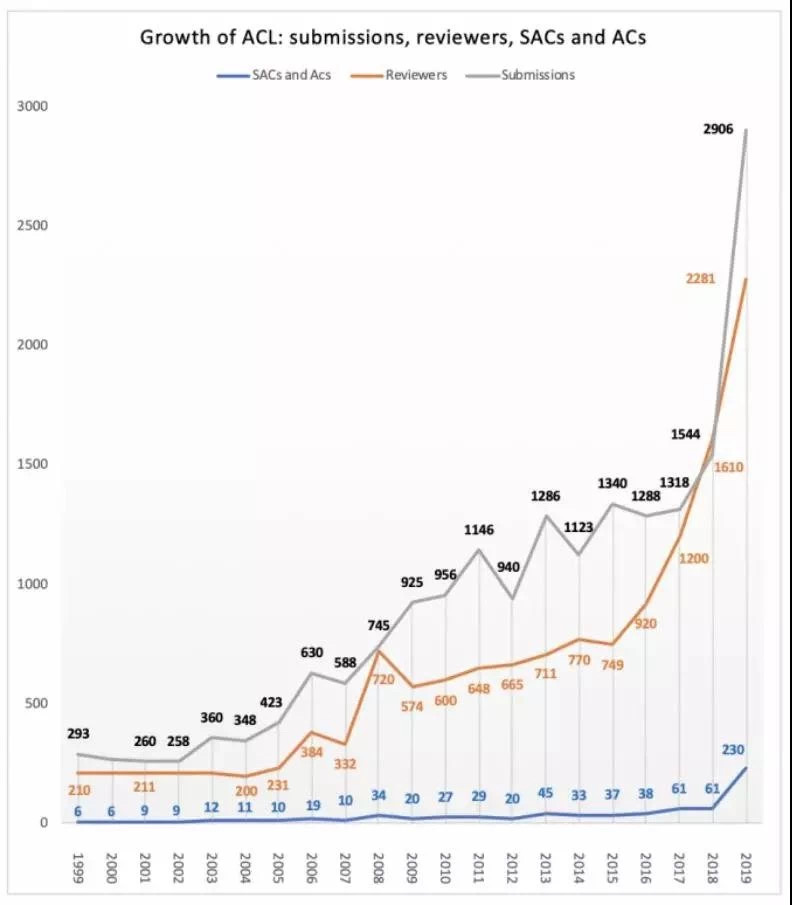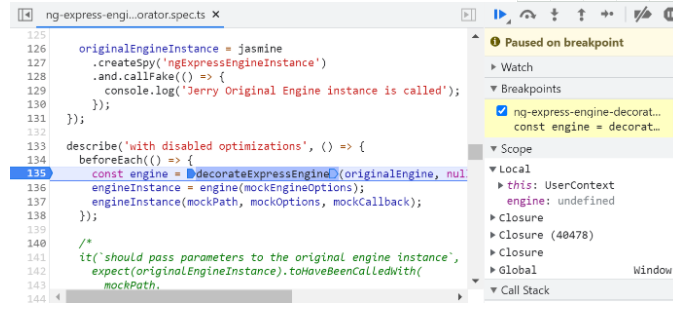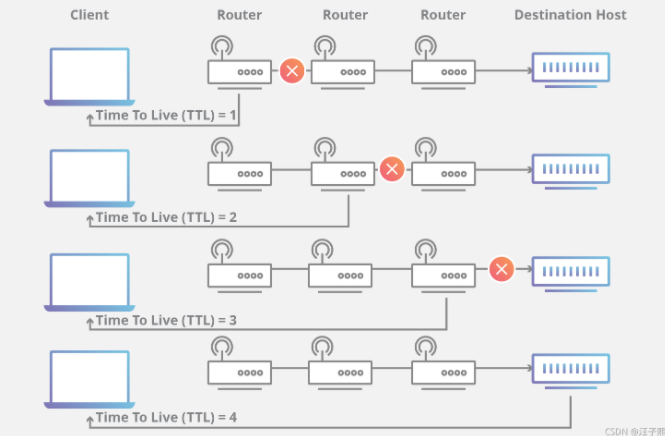# 堆排序

+关注继续查看

### 起源

1991年计算机先驱奖获得者、斯坦福大学计算机科学系教授罗伯特·弗洛伊德(Robert W．Floyd）和威廉姆斯(J．Williams）在1964年共同发明了著名的堆排序算法（ Heap Sort )

### “堆”定义

n个关键字序列Kl，K2，…，Kn称为（Heap），当且仅当该序列满足如下性质（简称为堆性质）：
(1)ki<=k(2i）且ki<=k(2i+1)(1≤i≤ n），当然，这是小根堆，大根堆则换成>=号。//k(i）相当于二叉树非叶结点，K(2i）则是左孩子，k(2i+1）是右孩子

【例】关键字序列（10，15，56，25，30，70）和（70，56，30，25，15，10）分别满足堆性质（1）和（2），故它们均是堆，其对应的完全二叉树分别如小根堆示例和大根堆示例所示。

### 堆排序

（1）用大根堆排序的基本思想
① 先将初始文件R[1..n]建成一个大根堆，此堆为初始的无序区
② 再将关键字最大的记录R（即堆顶）和无序区的最后一个记录R[n]交换，由此得到新的无序区R[1..n-1]和有序区R[n]，且满足R[1..n-1].keys≤R[n].key
③由于交换后新的根R可能违反堆性质，故应将当前无序区R[1..n-1]调整为堆。然后再次将R[1..n-1]中关键字最大的记录R和该区间的最后一个记录R[n-1]交换，由此得到新的无序区R[1..n-2]和有序区R[n-1..n]，且仍满足关系R[1..n-2].keys≤R[n-1..n].keys，同样要将R[1..n-2]调整为堆。
……

（2）大根堆排序算法的基本操作：
① 初始化操作：将R[1..n]构造为初始堆；
② 每一趟排序的基本操作：将当前无序区的堆顶记录R和该区间的最后一个记录交换，然后将新的无序区调整为堆（亦称重建堆）。

①只需做n-1趟排序，选出较大的n-1个关键字即可以使得文件递增有序。
②用小根堆排序与利用大根堆类似，只不过其排序结果是递减有序的。堆排序和直接选择排序相反：在任何时刻堆排序中无序区总是在有序区之前，且有序区是在原向量的尾部由后往前逐步扩大至整个向量为止

## 算法描述

### C语言描述

// array是待调整的堆数组，i是待调整的数组元素的位置，nlength是数组的长度
//本函数功能是：根据数组array构建大根堆
void HeapAdjust(int array[], int i, int nLength)
{
int nChild;
int nTemp;
for (nTemp = array[i]; 2 * i + 1 < nLength; i = nChild)
{
// 子结点的位置=2*（父结点位置）+ 1
nChild = 2 * i + 1;
// 得到子结点中较大的结点
if (nChild < nLength - 1 && array[nChild + 1] > array[nChild])
++nChild;
// 如果较大的子结点大于父结点那么把较大的子结点往上移动，替换它的父结点
if (nTemp < array[nChild])
{
array[i] = array[nChild];
}else{
// 否则退出循环
break;
}
// 最后把需要调整的元素值放到合适的位置
array[nChild]= nTemp;
}
}
// 堆排序算法
void HeapSort(int array[],int length)
{
// 调整序列的前半部分元素，调整完之后第一个元素是序列的最大的元素
for (int i = length / 2 - 1; i >= 0; --i)
{
}
// 从最后一个元素开始对序列进行调整，不断的缩小调整的范围直到第一个元素
for (int i = length - 1; i > 0; --i)
{
// 把第一个元素和当前的最后一个元素交换，
// 保证当前的最后一个位置的元素都是在现在的这个序列之中最大的
Swap(&array, &array[i]);
// 不断缩小调整heap的范围，每一次调整完毕保证第一个元素是当前序列的最大值
}
}

#define MAX 100//数据元素的最大个数
typedef struct
{
int r[MAX];
int length;
}SqList;//定义一个线性表用于存放数据元素
{//已知L.r[s...m]中记录除L.r[s]外均满足堆的定义，本函数用于使L.r[s...m]成为一个大顶堆
int j;
int e=L.r[s];
for(j=2*s;j<=m;j*=2)
{
if(j<M&&L.R[J]<L.R[J+1]) ++j;
if(e>=L.r[j]) break;
L.r[s]=L.r[j];
s=j;
}
L.r[s]=e;
}
void HeapSort(SqList &L)
{//对顺序表L进行堆排序
int i,e;
for(i=L.length/2;i>0;i--)
for(i=L.length;i>1;i--)
{//将大顶堆的顶记录和最后一个记录相互交换
e=L.r;
L.r=L.r[i];
L.r[i]=e;
}
}

"筛选法"调整堆
R[low]的左、右子树（若存在）均已是堆，这两棵子树的根R[2low]和R[2low+1]分别是各自子树中关键字最大的结点。若R[low].key不小于这两个孩子结点的关键字，则R[low]未违反堆[性质，以R[low]为根的树已是堆，无须调整；否则必须将R[low]和它的两个孩子结点中关键字较大者进行交换，即R[low]与R[large](R[large].key=max(R[2low].key，R[2low+1].key））交换。交换后又可能使结点R[large]违反堆性质，同样由于该结点的两棵子树（若存在）仍然是堆，故可重复上述的调整过程，对以R[large]为根的进行调整。此过程直至当前被调整的结点已满足性质，或者该结点已是叶子为止。上述过程就象过筛子一样，把较小的关键字逐层筛下去，而将较大的关键字逐层选上来。因此，有人将此方法称为"筛选法"。

### Heapify函数算法实例

#include
#include
inline int LEFT(int i);
inline int RIGHT(int i);
inline int PARENT(int i);
void MAX_HEAPIFY(int A[],int heap_size,int i);
void BUILD_MAX_HEAP(int A[],int heap_size);
void HEAPSORT(int A[],int heap_size);
void output(int A[],int size);
int main()
{
FILE *fin;
int m,size,i;
fin = fopen("arrayin","r");
int* a;
fscanf(fin," %d",&size);
a = (int *)malloc(size + 1);
a=size;
for(i = 1;i <= size; i++ )
{
fscanf(fin," %d",&m);
a[i]= m;
}
HEAPSORT(a,a);
printf("$$The Result$$\n");
output(a,a);
free(a);
return 0;
}
inline int LEFT(int i)
{
return 2 * i;
}
inline int RIGHT(int i)
{
return 2 * i + 1;
}
inline int PARENT(int i)
{
return i / 2;
}
void MAX_HEAPIFY(int A[],int heap_size,int i)
{
int temp,largest,l,r;
largest = i;
l = LEFT(i);
r = RIGHT(i);
if ((l <= heap_size) && (A[l] > A[largest])) largest = l;
if ((r<= heap_size) && (A[r] > A[largest])) largest = r;
if (largest != i)
{
temp = A[largest];
A[largest] = A[i];
A[i]= temp;
MAX_HEAPIFY(A[],heap_size,largest);
}
}
void BUILD_MAX_HEAP(int A[],int heap_size)
{
int i;
for (i = heap_size / 2;i >= 1;i--) MAX_HEAPIFY(A,heap_size,i);
}
void HEAPSORT(int A[],int heap_size)
{
int i;
BUILD_MAX_HEAP(A,heap_size);
for (i = heap_size;i >= 2; i--)
{
int temp;
temp = A;
A = A[heap_size];
A[heap_size]= temp;
MAX_HEAPIFY(A,i-1,1);
}
}
void output(int A[],int size)
{
int i = 1;
FILE *out = fopen("resultin","w+");
for (; i <= size; i++)
{
printf("%d ",A);
fprintf(out,"%d ",A);
}
printf("\n");
}

### Pascal/Delphi描述

const
max=100;
type
arr=array[1..max] of integer;
var
a:arr;
i,n,temp:integer;
procedure heap(var r:arr;nn,ii:integer); //之所以重新定义r数组而不是在a数组上修改，是为了能同时操作不同的数组
var
x,i,j:integer;
begin
i:=ii;
x:=r[ii];//把待调整的节点值暂存起来。
j:=ii shl 1;//j 为 ii 的左孩子编号，初始时假设它比右孩子的值大。
while j<=nn do
begin
if (j+1<=nn) and (r[j]<r[j+1]) then //必须是j+1，因为j=nn时，j+1>nn，会影响已排过的节点！
inc(j);//j 为值大的那个孩子的节点编号。
if x<r[j] then//调整。
begin
r[i]:=r[j];
i:=j;
j:=i shl 1
end
else j:=nn+1//故意让 j 超出范围，终止循环。
end;
r[i]:=x;//调整到最终位置。
end;
begin
for i:=1 to n do
writeln;
for i:=n shr 1 downto 1 do
heap(a,n,i);//建立初始堆，且产生最大值 A。
for i:=n downto 2 do//将当前最大值交换到最终位置上，再对前 i-1 个数调整。
begin
temp:=a;
a:=a[i];
a[i]:=temp;
heap(a,i-1,1)
end;
for i:=1 to n do
write(a[i]:4)
end.

Pascal源代码
var
i,n:integer;
a:array[0..100] of integer;
procedure swap(var a,b:integer);
var t:integer;
begin t:=a;a:=b;b:=t;
end;
procedure heapsort(i,m:integer);
var t:integer;
begin
t:=i*2;
while t<=m do
begin
if (t<m) and (a[t]>a[t+1]) then inc(t);
if a[i]>a[t] then begin swap(a[i],a[t]);i:=t;t:=2*i; end
else break;
end;
end;
begin
for i:=1 to n do read(a[i]);
for i:=n div 2 downto 1 do heapsort(i,n);
for i:=n downto 2 do
begin
write(a,' ');
a:=a[i];
heapsort(1,i-1);
end;
writeln(a);
end.

### JAVA描述

public class HeapSortTest {
/*
* 将数组调整为小根堆，即由小到大排序
*/
public static int[] heap = new int[] { 1,3,7,5,2,8,4,6,10,9};
public static void main(String[] args) {
int temp;
/*
* 创建堆（对该堆进行简单的排序）
*/
CreateHeap();
for (int i = heap.length - 1; 0 < i; i--) {
temp = heap;
heap = heap[i];
heap[i] = temp;
/*
* 展示每次排序后的结果
*/
for (int j = 0; j < heap.length; j++) {
System.out.print(heap[j] + " ");
}
System.out.println();//换行
/*
* 从堆顶进行调整，使未排序堆中最大关键字到堆顶
*/
}
}
/*
* 调整堆使其堆顶为未排序堆中最大关键字
*/
public static void AdjustHeap(int location,int unSortlength) {
int temp;
int tempLoc;
/*
* 确保左右节点存在
*/
if ((tempLoc = (location + 1) * 2) < unSortlength) {
/*
* 判断左右节点大小
*/
if (heap[tempLoc] >= heap[tempLoc - 1]) {
/*
* 判断父节点与子节点的大小，若父节点小，则与大的子节点换位
*/
if (heap[location] < heap[tempLoc]) {
temp = heap[location];
heap[location] = heap[tempLoc];
heap[tempLoc] = temp;
/*
*递归法对换位后的子节点及其子节点进行调整
*/
}
} else {
/*
* 左节点大于右节点
*/
if (heap[location] < heap[tempLoc - 1]) {
temp = heap[location];
heap[location] = heap[tempLoc - 1];
heap[tempLoc - 1] = temp;
/*
* 递归法对换位后的子节点及其子节点进行调整
*/
}
}
}
/*
* 确保左节点存在
*/
else if ((tempLoc = (location + 1) * 2 - 1) < unSortlength) {
/*
* 与左节点进行比较
*/
if (heap[location] < heap[tempLoc]) {
/*
*左子节点大于父节点，将两者进行换位
*/
temp = heap[location];
heap[location] = heap[tempLoc];
heap[tempLoc] = temp;
}
}
}
/*
* 创建堆（对该堆进行简单的排序）
*/
public static void CreateHeap() {
for (int i = heap.length - 1; i >= 0; i--) {
}
}
}

### c++描述（降序）

/* 这是经过优化的代码。一般都用大根堆，但是如果用小根堆实现，每次都将最小的元素放到最后一个，可以省一半空间。用c数组是因为我写一道题目需要给c数组排序……直接粘过去，偷懒一下 &^_^& */
#include<iostream>
using namespace std;
int c;
int n,m;
void check(int i)
{
int min;
if(m>=i*2+1) //不能用n，因为需要排序的元素个数在不断变化
{
if(c[i*2+1]<c[i*2])
min=i*2+1;
else
min=i*2;
if(c[min]<c[i])
{
swap(c[i],c[min]);
check(min);
}
} //这几行也可以换成判断然后交换c[i]和c[i*2+1]，又可以省去几行。哈哈。
else
if(m>=i*2 && c[i*2]<c[i])
{
swap(c[i*2],c[i]);
check(i*2);
}
}
//check函数用来维持小根堆
void tree()
{
m=n;
for(int i=m/2;i>=1;i--)
check(i);
while(m!=1)
{
swap(c,c[m]); //将最小的元素放到最后，这个元素以后的元素就是有序的，不用参加排序了
m--;
check(1);
}
}
//tree函数用来建堆
int main()
{
cin>>n;
for(int i=1;i<=n;i++)
cin>>c[i];
tree();
for(i=1;i<=n;i++)
cout<<c[i]<<' ';
cout<<endl;
system("pause"); //为了方便观察结果才写的这一行，提交题目的时候一定要删掉！！！
return 0;
}

### AAuto语言描述

io.open();//打开控制台
/*
*-------------------------------------------------------
* 堆排序（ 原地排序 )
*-------------------------------------------------------
*/
/*


 
∧ ∧
   

heap = 29;
heap = 19;
heap = 18;
heap = 9;
heap = 8;
heap = 7;
heap = 6;

29
19 18
∧ ∧
9 9 7 6

*/
getParentIndex = function(i){
//二叉堆的父子索引总是以2倍的形式递增、堆左边索引是2的n次方。
return math.floor(i/2)//所以除2总是能向上倒退一层到父节点的索引。
}
getLeftNodeIndex = function(i){
//父层到子层，总是一变二，所以索引也增加2倍
return 2*i
}
getRightNodeIndex = function(i){
return 2*i+1
}
/*

*/
max_heapify = function(array,i){
var l = getLeftNodeIndex(i);
var r = getRightNodeIndex(i);
var largest;
if( ( l<=array.heapsize ) and( array[l] > array[i]) )
largest = l;//左子树比较大
else{
largest = i;//父节点比较大
}
if( ( r<=array.heapsize ) and( array[r] > array[largest]) )
largest = r;//右子树比较大
//如果array[i]小于其子女，则调节array[i]，使之下降。
if( largest != i){
var exchange = array[i]
array[i] = array[largest]
array[largest] = exchange;
max_heapify(array,largest);//现在子树largest是最大的了
}
}
/*

#A的父节点为 math.floor(#A/2) ，而且是最后一个父节点。而之后都是叶节点。

*/
//建堆
build_max_heap = function(array){
array.heapsize = #array;
for(i=math.floor(#array/2);1;-1){
max_heapify(array,i);
}
}
/*

io.print( table.tostring(array) ) //输出看一下，整体上仍然是乱的
*/
/*

*/
//那么下面我们要利用建好的堆来排序
heap_sort = function(array){
build_max_heap(array)//建堆
//现在array是根节点了，并且一定是最大的
//我们把最大的放在数组最右侧，后把最右侧较小的元素放到根节点来破坏二叉堆，再用max_heapify来修复堆取到下一个最大的数
//不断循环此过程，不断的将最大的元素移到右侧，而堆向左收缩，就达到了排序的效果
for(sorted=#array;2;-1){
var max = array
array = array[sorted] //把小的移到二叉堆的顶部来破坏堆定义
array[sorted] = max;//把最大的移到右侧已排序的数组中
array.heapsize--;
max_heapify(array,1);//修复二叉堆根节点，取下一个最大数
}
}
//终于排序好了输出看一下
io.print("----------------")
io.print("堆排序")
io.print("----------------")
array ={2;46;5;17;1;2;3;99;12;56;66;21};
heap_sort(array)
//输出结果
for(i=1;#array;1){
io.print( array[i] )
}
/*

*/
execute("pause") //按任意键继续
io.close();//关闭控制台

### js语言描述

//5000个无序随机里取最大的50个数
//定义
var heap={};
//大数组，从1-1000000取5000个随机数
heap.arr=[];
for(var i=0;i<5000;i++){
heap.arr.push(Math.floor(1000000*Math.random())+1);
}
//取前50个数作为堆
heap.arrTemp=heap.arr.slice(0,50).sort(function(a,b){
return a-b;
});
//无序数组里挨个与堆的最小数判断，大则入堆，堆重新排序，返回50个最大的数
heap.j=50;
(function(){
while(heap.j<5000){
if(heap.arr[heap.j]>heap.arrTemp){
heap.arrTemp.splice(0,1,heap.arr[heap.j]);
heap.arrTemp.sort(function(a,b){
return a-b;
});
}
heap.j++;
}
return heap.arrTemp;
})();ACL 2019 接收论文榜单发布，我们做了可视化分析

6 0SAP 电商云 Spartacus UI SSR 里 engine 和 engine instance 的区别
SAP 电商云 Spartacus UI SSR 里 engine 和 engine instance 的区别
3 07 05 04 0Edge Cache(边缘缓存) 的概念
Edge Cache(边缘缓存) 的概念
4 04 0HTTP 头部字段 Cache Control max-age = 0 和 no-cache 的区别
HTTP 头部字段 Cache Control max-age = 0 和 no-cache 的区别
3 04 06 0+关注

301

311

+ 订阅

《SaaS模式云原生数据仓库应用场景实践》

《看见新力量：二》电子书